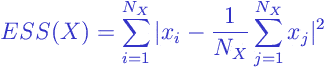# Glossary

Ward´s linkage is a method for hierarchical cluster analysis . The idea has much in common with analysis of variance (ANOVA). The linkage function specifying the distance between two clusters is computed as the increase in the “error sum of squares” (ESS) after fusing two clusters into a single cluster. Ward´s Method seeks to choose the successive clustering steps so as to minimize the increase in ESS at each step.

The ESS of a setofvalues is the sum of squares of the deviations from the mean value or the mean vector ( centroid ). For a setthe ESS is described by the following expression:where

•is the absolute value of a scalar value or the norm (the “length”) of a vector.

Mathematically the linkage function – the distance between clustersand– is described by the following expressionwhere

•is the combined cluster resulting from fusion clustersand;
•is the error sum of squares describe above.

Browse Other Glossary Entries

## Test Yourself

Planning on taking an introductory statistics course, but not sure if you need to start at the beginning? Review the course description for each of our introductory statistics courses and estimate which best matches your level, then take the self test for that course. If you get all or almost all the questions correct, move on and take the next test.

### Data Analytics

Considering becoming adata scientist, customer analyst or our data science certificate program?

Analytics Quiz

Statistics Quiz

### Statistics

Looking at statistics for graduate programs or to enhance your foundational knowledge?

Statistics 1 Quiz

Regression Quiz

Regression Quiz

### Biostatistics

Entering the biostatistics field? Test your skill here.

Biostatistics Quiz

Statistics 2 Quiz

### Stay Informed

Our Blog

Read up on our latest blogs

Certificates

Courses

Find the right course for you